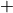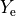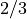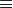# Parameter List by Name¶

Name

Number

Format

Unit

Synopsis

angit

113

float

-

Total momentum of inertia of the star.

anglint

80

float

erg*sec

Initial angular momentum.

angloss

111

float

-

Total angular momentum removed from problems by cutsurf.

anglossx

154

float

erg*sec

X-component of angular momentum removed from problems by cutsurf.

anglossy

155

float

erg*sec

Y-component of angular momentum removed from problems by cutsurf.

anglossz

156

float

erg*sec

Z-component of angular momentum removed from problems by cutsurf.

anglt

81

float

erg*sec

Current total angular momentum.

angltx

151

float

erg*sec

X-component of current total angular momentum.

anglty

152

float

erg*sec

Y-component of current total angular momentum.

angltz

153

float

erg*sec

Z-component of current total angular momentum.

anglwnd

112

float

-

Total angular momentum lost due to wind.

anglwndx

157

float

-

X-component of angular momentum lost due to wind.

anglwndy

158

float

-

Y-component of angular momentum lost due to wind.

anglwndz

159

float

-

Z-component of angular momentum lost due to wind.

capeff

87

float

cm**2/g

chi0

65

float

-

Neutrino flux parameter. Set by the nflux card in the LINK file (see Link Input File).

chi2

69

float

-

Neutrino flux parameter. Set by the nflux card in the LINK file (see Link Input File).

chil

67

float

-

Neutrino flux parameter. Set by the nflux card in the LINK file (see Link Input File).

decmass

130

float

g

Total mass removed from bottom and not added to substrate.

delmass

128

float

g

Mass lost in last time step in.

dmacc

125

float

g

Mass accreted in last time step in.

dmdec

131

float

g

Mass removed in last time step.

dt

1

float

sec

KEPLER timestep.

dtold

3

float

sec

KEPLER timestep used for the previous cycle.

dtsub

58

float

-

Length of a BURN co-processing/BURN ion convection subcycle (see nsubcycm (p 264)).

dvacc

137

float

cc

Volume change due to mass accretion.

dvdev

138

float

cc

Volume change due to mass decretion (from bottom).

dvloss

136

float

cc

Volume change due to mass loss.

enc

37

float

erg

Energy check.

encd

45

float

erg/sec

Total rate of change in the energy check.

enes

36

float

erg

Total non-neutrino energy that has so far escaped from the star’s surface.

enesd

44

float

erg/sec

Total rate of energy escape from the star (in photons).

enhd

95

float

-

Rate of change of thermal (“heat”) energy content.

eni

31

float

erg

Total internal energy.

enid

39

float

erg/sec

Rate of change in the total internal energy.

eniloss

107

float

-

Internal energy removed from problems by cutsurf.

enini

26

float

erg

Total initial internal energy.

enink

27

float

erg

Total initial kinetic energy.

eninp

28

float

erg

Total initial potential energy.

eninr

78

float

erg

Initial rotational energy.

enint

29

float

erg

Total initial energyenini (q 26)enink (q 27)eninp (q 28).

enk

32

float

erg

Total kinetic energy.

enkd

40

float

erg/sec

Rate of change in the total kinetic energy.

enkloss

108

float

-

Kinetic energy removed from problems by cutsurf.

enn

84

float

erg

Total neutrino energy lost from the star.

ennidec

145

float

erg

Energy depositied bydecay.

ennidecd

146

float

erg/sec

Energy deposition rate bydecay.

enp

33

float

erg

Total potential energy.

enpd

41

float

erg/sec

Rate of change in the total potential energy.

enpist

85

float

erg

Total energy input by the piston.

enpistd

86

float

erg/sec

Energy input rate by the piston.

enploss

109

float

-

Potential energy removed from problems by cutsurf.

enpuls

143

float

erg

Energy depositied by pulsar.

enpulsd

144

float

erg/sec

Energy deposition rate by pulsar.

enr

79

float

erg

Current rotational energy.

enrd

102

float

erg/sec

Rate of change of rotational energy during last step.

enrloss

110

float

-

Rotational energy removed from problems by cutsurf.

ensc

30

float

erg

Total energy deposited so far from input “source”. See xlum0 (p 62).

enscd

38

float

erg/sec

Rate of energy deposition by input “source”. See xlum0 (p 62).

ent

34

float

erg

Total current energyeni (q 31)enk (q 32)enp (q 33).

entd

42

float

erg/sec

Rate of change in the total energy.

entloss

106

float

-

Total energy removed from problems by cutsurf.

env

139

float

erg

Total viscous energy dissipated.

envc

141

float

erg

Total viscous energy dissipation check.

envcd

142

float

erg/sec

Viscous energy dissipation rate check in last time step.

envd

140

float

erg/sec

Viscous energy dissipation rate in last time step.

enxheat

148

float

erg

Total energy deposition by extra heating.

enxheatd

147

float

erg/sec

Total rate of energy deposition by extra heating.

epro

35

float

erg

Total net energy produced so far by nuclear reactions less neutrino losses.

eprod

43

float

erg/sec

Total rate of nuclear energy production less neutrino losses.

eprodw

120

float

erg/sec

WIMP energy deposition rate in star.

eprodwx

122

float

erg/sec

WIMP annihilation luminosity emph{outside} star.

eprow

121

float

erg

Total energy deposited in star by WIMP annihilation.

eprowx

123

float

erg

Total energy produced by wimps OUTSIDE star by WIMP annihilation.

fallback

99

float

-

Total fallback mass.

fbrate

98

float

-

Fallback accretion rate.

fcl

11

float

-

Maximum fractional zonal luminosity change since the last iteration.

fcr

5

float

-

Maximum fractional zonal radius change since the last iteration.

fct

6

float

-

Maximum fractional zonal temperature change since the last iteration.

fluxnu0

63

float

-

Neutrino flux parameter. Set by the nflux card in the LINK file (see Link Input File).

fluxnua

64

float

-

Neutrino flux parameter. Set by the nflux card in the LINK file (see Link Input File).

fluxnub

66

float

-

Neutrino flux parameter. Set by the nflux card in the LINK file (see Link Input File).

fluxnuc

68

float

-

Neutrino flux parameter. Set by the nflux card in the LINK file (see Link Input File).

h1init

101

float

-

Initial central h1 mass fraction.

he4init

133

float

-

Initial He4.

idmptime

72

integer

-

Integer index of the next dump time (dump cycle number in subroutine DUMPQ).

idtcon

13

integer

-

Symbol of the ion most limiting the current KEPLER timestep.

ilastpl

103

integer

-

”Time-of-day” seconds of last plot.

imax

14

integer

-

Total number of ions in the APPROX network (usually 19).

imaxb

53

integer

-

Number of isotopes in the currently specified BURN network (typically 149).

isebkup

94

integer

-

Number if ISE non-convergence backups in subroutine SDOT.

iter

7

integer

-

Number of iterations taken for convergence.

iterbar

160

integer

-

Current iteration counter for problem termination.

itert

19

integer

-

Total number of iterations since problem generation.

itimed

105

integer

-

Time/date when dump was generated.

itimeg

104

integer

-

Time/date when problem was generated.

jacc

127

integer

-

Accretion zone.

jaccemx

149

integer

-

jbmax

57

integer

-

Maximum zone number for which BURN co-processing is now being done.

jfcl

22

integer

-

Zone with largest fractional luminosity change since the last iteration.

jfcr

20

integer

-

Zone with largest fractional radius change since the last iteration.

jfct

21

integer

-

Zone with largest fractional temperature change since the last iteration.

jlm

56

integer

-

Number of zones at the end of the last KEPLER cycle.

jloss

126

integer

-

Mass losing zone.

jm

2

integer

-

Number of zones.

jpist

59

integer

-

Index of the piston position now being used (see :ref:’link’ and cycle).

jpistm

60

integer

-

Total number of piston positions specified in the LINK file (see Link Input File).

locqnext

76

integer

-

Location of the next open track in the current ‘qq’ dump file (see subroutine DUMPQ).

mncbkup

92

integer

-

Number mass non-conservation backups in subroutine SDOT for APPROX network.

nacbkup

93

integer

-

Number of excess abundance change backups in subroutine SDOT for APPROX network.

nburnz

51

integer

-

Number of zones co-processed by BURN during current KEPLER cycle.

nburnzt

52

integer

-

Total number of zones co-processed by BURN since problem generation.

ncyc

4

integer

-

KEPLER cycle number.

ncycb

49

integer

-

Current cycle number used by the BURN coprocessor (should be same as ncyc).

ncycbt

50

integer

-

Number of cycles since BURN co-processing was initiated (usually = ncyc).

ncycr

100

integer

-

Cycle of problem generation or last restart.

ndatl

77

integer

-

Current number of specified ‘look’ variables (see subroutine READQ and ADDLOOK).

ndatq

75

integer

-

Current number of zonal edit variables to be dumped(see subroutines DUMPQ & DUMP).

ngbkup

91

integer

-

Number of negative abundance backups in subroutine SDOT for APPROX network.

ninvl

90

integer

-

Number of BURN matrix inversions in last cycle.

nnet

15

integer

-

Number of ion networks specified.

nnetb

54

integer

-

Number of specified BURN isotopic networks (usually 1).

nreac

18

integer

-

Number of nuclear reactions edited (ions only – usually 29).

numit

16

integer

-

Total number of ions specified in the generator (usually 19).

numitb

55

integer

-

Total number of isotopes specified in the BURN generator (typically 149).

nye

70

integer

-

Index of the LINK-specified Ye now being utilized (see Link Input File and subroutine sdot).

nyem

71

integer

-

Total number of ye-card-specifiedvalues in the LINK file (see Link Input File).

nylib

73

integer

-

Number of distinct mass coordinates used so far in describing zoning (ylib entries).

nylib0

74

integer

-

Number of ylib entries (see nylib (q 73)) at the end of the last qq dump cycle.

pboundac

96

float

erg/cc

Boundary pressure due to accretion.

pboundat

150

float

erg/cc

Atmosphere surface boundary pressure resulting from use of isurf (p 523).

89

float

cm

Radius outside of which no convection is allowed.

47

float

cm

Photospheric radius corresponding to radius withoptical depth.

s0exp

24

float

erg/g

Neutrino deposition coefficient derived from the values of parameters jshell0 (p 93), jshell1 (p 94), and eexplode (p 95) and the current zonal of the star.

snuc

23

float

erg/g/sec

Current nuclear energy generation rate from last call to subroutine SDOT.

tbounce

62

float

sec

Core bounce time. Set by the nflux card in the LINK file (see Link Input File).

teff

48

float

K

Effective surface temperature at radius (q 47).

tfcl

12

float

-

Total maximum fractional zonal luminosity change since the last iteration.

tfcr

9

float

-

Total maximum fractional zonal radius change during current cycle.

tfct

10

float

-

Total maximum fractional zonal temperature change during current cycle.

told

8

float

sec

Time at the beginning of the last timestep.

totm

17

float

g

Current total amount of mass in the problem (incl. summ0 (p 61)).

velnegm

97

float

-

This quantity is for fallback test only.

velocty0

61

float

cm/sec

Velocity of inner problem boundary due to specified piston (see Link Input File).

wimpalum

119

float

erg/sec

Total WIMP annihilation luminosity as computed by WIMP subroutine in.

116

float

cm

wimparat

118

float

sec

WIMP annihilation rate (two WIMPs annihilate in one annihilation “event”).

wimpateq

117

float

sec

WIMP capture & annihilation equilibrium time scale.

wimpcrsd

115

float

1/sec

WIMP spin-emph{depenedent} capture rate.

wimpcrsi

114

float

1/sec

WIMP spin-emph{indepenedent} capture rate.

135

float

erg/sec

xlum

46

float

erg/sec

Surface luminosity in electromagnetic radiation (enesd).

xlumeff

161

float

erg/sec

xlumn

25

float

erg/sec

Neutrino luminosity.

xmacc

124

float

g

Total amount of mass accreted in.

xmacrate

132

float

g/sec

Accretion rate.

xmdec

129

float

g

Total mass removed from bottom.

xmlossr

82

float

g/sec

Current mass loss rate.

xmlost

88

float

g

Mass lost in the “wind”.

zfak

83

float

-

Computed metallicity multiplier on mass loss rate.

zinit

134

float

-

Initial metallicity.